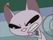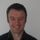cancel
Showing results for
Did you mean:New Member

## Join all sheets in one excel after transforming them individually

have an excel file with multiple sheets which I want first to transform them individually (remove 3 top rows, promote headers, remove top row, unpivot columns that are not column1=), and then annex them all together.

Is that possible? I tried to use a transform from combining files, but I just get an error becasue I'm trying to transform tables instead of a binary.

Sam

1 ACCEPTED SOLUTIONSolution Sage

hi, @snaise

1. Create a custom function with all your transformations steps.

3. Filter [Kind] column to get sheets only.

4. Apply your function to [Data] column: Table.TransformColumns(Source, each {"Data", my_function})

5. Table.Combine [Data] column

So

``````let
Source = Excel.Workbook(File.Contents("C:\Book1.xlsx"), null, true),
filter_sheets = Table.SelectRows(Source, each ([Kind] = "Sheet")),
my_function = (t as table) =>
let
a = Table.RemoveFirstN(t, 3),
c = Table.RemoveFirstN(b, 1),
d = Table.UnpivotOtherColumns(c, {"Column1"}, "Attribute", "Value")
in d,
transform = Table.TransformColumns(filter_sheets, {"Data", my_function}),
combine = Table.Combine(transform[Data])
in
combine``````

3 REPLIES 3Solution Sage

hi, @snaise

1. Create a custom function with all your transformations steps.

3. Filter [Kind] column to get sheets only.

4. Apply your function to [Data] column: Table.TransformColumns(Source, each {"Data", my_function})

5. Table.Combine [Data] column

So

``````let
Source = Excel.Workbook(File.Contents("C:\Book1.xlsx"), null, true),
filter_sheets = Table.SelectRows(Source, each ([Kind] = "Sheet")),
my_function = (t as table) =>
let
a = Table.RemoveFirstN(t, 3),
c = Table.RemoveFirstN(b, 1),
d = Table.UnpivotOtherColumns(c, {"Column1"}, "Attribute", "Value")
in d,
transform = Table.TransformColumns(filter_sheets, {"Data", my_function}),
combine = Table.Combine(transform[Data])
in
combine``````New Member

The question is that is an excel file were they add each weeks info in a new tab, so do it individually doesn't work for me. I'm looking for a way were you can deal with them as files you combine, with one generic transformation for all of them.Super User

Hi @snaise

Yes this is possible.  You just need to load each sheet individually with its own query, do your transformations, then combine the queries.

To load a sheet you'd use the Excel Workbook connector then choose the sheet, the query looks like this

``````let
Source = Excel.Workbook(File.Contents("D:\temp\Book1-3-pgt2.xlsx"), null, true),
Source_Sheet = Source{[Item="Source",Kind="Sheet"]}[Data],
in

To combine the sheets you can either merge them or append them.  I'm not sure what you want but happy to help if you need it.

This article also descibes how to combine Excel worksheets with power query.

Regards

Phil

If I helped you, click on the Thumbs Up to give Kudos.

Proud to be a Super User!